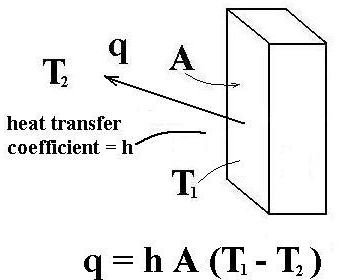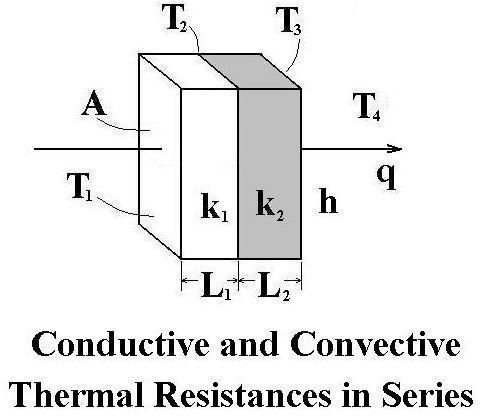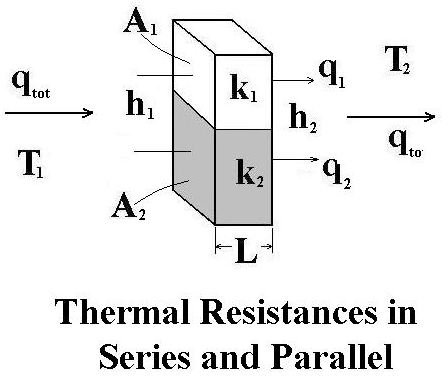# Thermal Conduction/Thermal Convection Heat Transfer Calculations Using Thermal Resistances

Page content

## Introduction

Fourier’s Law of Conduction is typically used for thermal conduction calculations and Newton’s Law of Cooling is used for thermal convection calculations. Thermal conduction and thermal convection seldom take place in isolation, however. Often conduction, convection and/or radiation take place together. For heat transfer calculations involving convection, conduction, and/or radiation combined, it’s convenient to use thermal resistances for each type of heat transfer in calculations. This article will cover the use of thermal resistances in combined conduction and convection heat transfer calculations, including an example problem. The convection thermal resistance is calculated from the convection heat transfer coefficient and the conduction thermal resistance is calculated from the thermal conductivity.

## Fourier’s Law of Conduction

As mentioned above, Fourier’s law of heat conduction and Newton’s law of cooling are typically used for heat transfer calculations involving thermal conduction or thermal convection, when there is enough information about each individual heat transfer process.

Fourier’s Law for Heat Conduction will be presented in this section. Newton’s Law of Cooling will be presented in the next section. Then the procedure for rearranging the equations to make them useful for combined convection conduction heat transfer will be considered.

Fourier’s Law of Thermal Conduction is: q = kA(ΔT/L), where

q = the rate of conduction heat transfer in Btu/hr,

k = thermal conductivity of the material through which thermal conduction is taking place, in Btu/hr-oF-ft,

A = area perpendicular to heat flow, ft2,

ΔT = the temperature difference that is driving the heat transfer, oF,

L = the distance through which conduction heat transfer is taking place, ft.

These parameters are illustrated for conduction heat transfer in the diagram above.

## Newton’s Law of Cooling

Newton’s Law of Cooling is used for both natural convection (fluid flow due to temperature induced fluid density variation) and forced convection (fluid flow caused by external forces such as wind, fans, pumps, etc.). Following is the normal form of Newton’s law of cooling as it is used for convection heat transfer:q = h A ΔT, where

q = the rate of convection heat transfer in Btu/hr,

h = the convection heat transfer coefficient in But/hr-ft2-oF,

A = the area perpendicular to heat flow in ft2,

ΔT = the temperature difference which is driving the convective heat transfer in oF.

These parameters are illustrated for convection heat transfer in the diagram at the right.

For downloadable Excel spreadsheets to calculate forced convection heat transfer coefficients for a variety of common forced convection heat transfer configurations, see the article, “Calculation of Forced Convection Heat Transfer Coefficients with Excel Spreadsheets.”

## Thermal Resistance for Conduction and Convection Heat TransferFourier’s Law of Conduction and Newton’s Law of Cooling can each be rearranged into the form:flow rate = (driving force)/resistance, where q is the flow rate (of heat), ΔT (or T1 - T2) is the driving force for the heat flow, and the denominator on the right hand side is the thermal resistance to heat flow in each of the following equations:

Conduction heat transfer: q = ΔT/(L/kA) ;

thus {Rcond = L/kA is the resistance to conductive heat transfer}

Convective heat transfer: q = ΔT/(1/hA) ; thus {Rconv = 1/hA is the resistance to convective heat transfer}

With these equations for conductive and convective thermal resistance available, analysis of combined convection/conduction heat transfer taking place in series or in parallel can be done using the same equations used for electrical resistances in series or parallel, as follows:

Resistances R1 and R2 in series (either electrical or thermal resistances): Rtot = R1 + R2

Resistances R1 and R2 in parallel (either electrical or thermal resistances): 1/Rtot = 1/R1 + 1/R2 or Rtot = R1R2/(R1 + R2)

The concept of convection/conduction thermal resistances in series and in parallel are illustrated in the figures above in this section.

## Example Thermal Conduction/Convection Calculation

Problem Statement: Consider heat flow through a two layer wall as shown in the diagram on the left above in the previous section. Given:

T1 = inside wall surface temperature = 250oF;

T4 = outside air temerature = 40oF;

L1 = 0.8 ft; L2 = 1.2 ft;

h = convection heat transfer coeffient at the outside of the wall = 3 Btu/hr-ft2-oF;

k1 = 0.70 Btu/hr-ft-oF; and k2 = 1.1 Btu/hr-ft-oF.

Calculate the rate of heat flow through the wall per unit area, q/A in Btu/hr-ft2.

Solution: The rate of heat flow will equal the total temperature difference divided by the sum of the three thermal resistances (since the three thermal resistances are in series):

q = (T1 - T4)/[L1/k1A + L2/k2A + 1/hA]; This can also be written as:

q/ A = (T1 - T4)/[L1/k1 + L2/k2 + 1/h] =(250 - 40)/(0.8/0.7 + 1.2/1.1 + 1/3) Btu/hr-ft2 = 81.8 Btu/hr-sq.ft.

References for Further Information:

1. Bengtson, Harlan H, Fundamentals of Heat Transfer, an online continuing education course for engineering PDH credit

2. Incropera, F.P., DeWitt, D.P, Bergman, T.L., & Lavine, A.S., Fundamentals of Heat and Mass Transfer, 6th Ed., Hoboken, NJ, John Wiley & Sons, (2007).

3. Lienhard, J.H, IV and Lienhard, J.H. V, A Heat Transfer Textbook: A Free Electronic Textbook

## This post is part of the series: Heat Transfer Fundamentals

Learn about conduction, convection, and radiation, the three mechanisms for heat transfer, including example calculations. Conduction heat transfer is of interest mostly in solids. Convection heat transfer is due to a moving fluid. Heat can also be transferred by electromagnetic radiation.